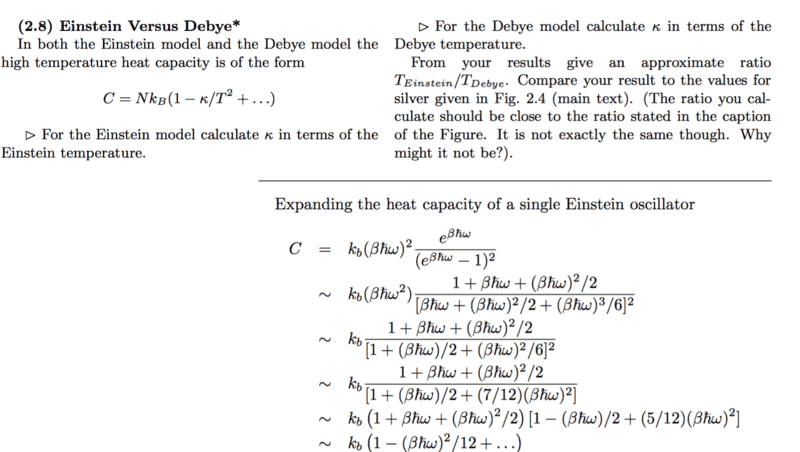# Confusing expansion

• I
I came across the following working in my notes and would like some help understanding how the step was done. Many thanks in advance!

The following is the working, and we assume that ##\beta## is small
$$\frac{1}{1+ \beta \hbar \omega /2 + (7/12)(\beta \hbar \omega)^2 +...} \approx 1 - (\beta \hbar \omega)/2 + (5/12) (\beta \hbar \omega)^2 +...$$

I thought of trying the Taylor expansion for ##1/(1-x)## where the ##x## was everything apart from the "1" in the reciprocal term on the LHS but it doesn't seem to work.

DrClaude
Mentor
I thought of trying the Taylor expansion for ##1/(1-x)## where the ##x## was everything apart from the "1" in the reciprocal term on the LHS but it doesn't seem to work.
That should be the right procedure, but it does give a slightly different result. Can you give a source for the equation you quoted?

Homework Helper
Gold Member
## \frac{1}{1-x}=1+x+x^2+x^3+... ##. In this case you need to consider the ## x^2 ## term as well.

fresh_42
Mentor
2021 Award
If we multiply the two expressions, we get ##1 \approx 1+ O(\beta^2)## so my suspicion is, that a) this expression is good enough for what is to come and b) the expression on the right is in some meaning more helpful than the "correct" formula. In short: it suffices and fits the goal.

Homework Helper
Gold Member
I get a ## -\frac{1}{3} ## instead of the ## +\frac{5}{12} ##. ## \\ ## Edit: I double-checked my arithmetic/algebra, and even worked a numerical example, letting ## \beta \hbar \omega=.02 ##. I think they goofed. It is something they should get right, but textbooks do contain errors on occasion.## \\ ## And you do get a +5/12 on the quadratic term if there is no 2 in the denominator of the ## \beta \hbar \omega ## term. I think it is probably an honest mistake.

Last edited:
Thanks everyone for the clarification.

That should be the right procedure, but it does give a slightly different result. Can you give a source for the equation you quoted?

It was a solution to one of the problems in Steven Simon's "Oxford Solid State Basics", I'll attach an image below. The step I refer to is the third from bottom.#### Attachments

mjc123
Homework Helper
There is a mistake in step 3: expanding the square in the denominator leads to a term of βħω, not βħω/2. Then the term βħω/2 in step 4 is wrong, but the 5/12 is right.

•Homework Helper
Gold Member
Looks like the final line is correct=even though they made a couple of errors previous to that.

•DrClaude
mfb
Mentor
Just a typo in one line. The author probably copied the previous line, adjusted it and forgot to remove the /2 there.

Another example where more context helps to spot the error.

Cheers guys, I should have been a bit more attentive, and also uploaded the image from the get-go. Apologies.

•